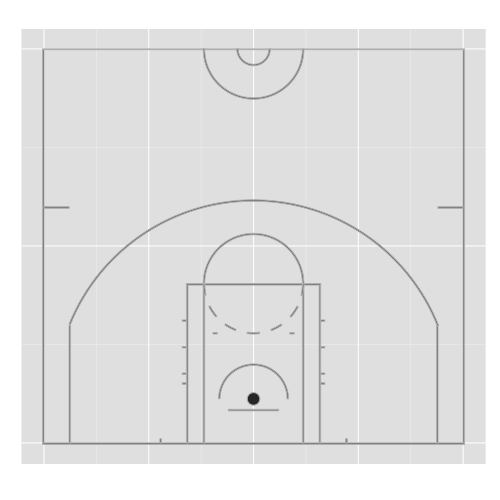Basketball court with ggplot2.

Following freakonometricstweet on basketball and big data, linking to this really cool javascript animation, as seen on @kirkgoldsberry‘s article on Grantland, I have spent some time drawing a basketball court in R. I have tried to be the most accurate possible, using this layout.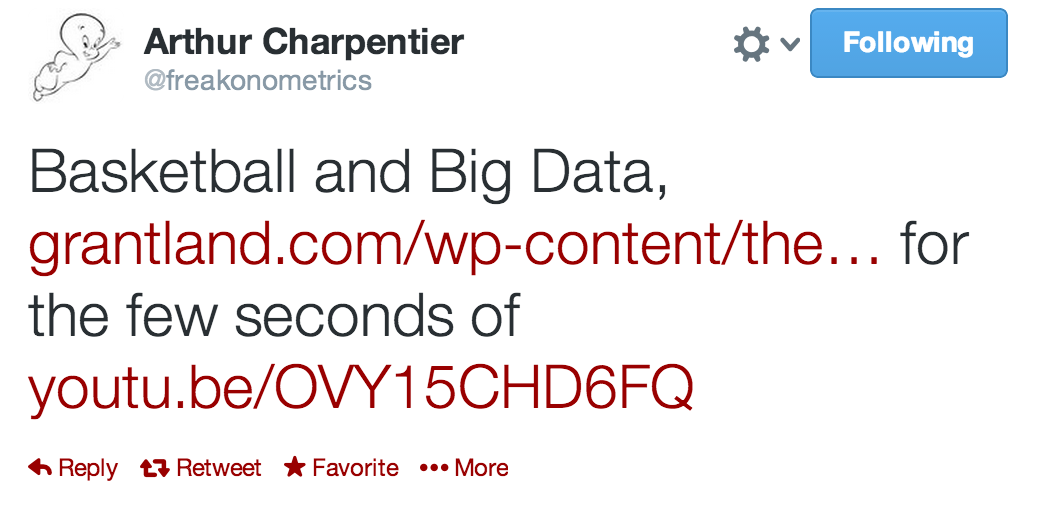First, I wanted to put some PNG images, as did Andy Woodruff and Kirk Goldsberry on the article on Grantland. Unfortunately it looked really ugly on a plot made with R (I bet there is a way, because nothing can be ugly with R, right?). So I decided to create a basketball court first, using ggplot2 (for a change).

Let’s have a look at the code. First, we need a function to draw circle because there is no “geom_circle” method (yet?). Hence, we have to be a bit dirty playing with that function.

# http://stackoverflow.com/questions/6862742/draw-a-circle-with-ggplot2
circle_fun <- function(center=c(0,0), diameter=1, npoints=500, start=0, end=2){
tt <- seq(start*pi, end*pi, length.out=npoints)
data.frame(
x = center + diameter / 2 * cos(tt),
y = center + diameter / 2 * sin(tt)
)
}


If we want to draw the whole court, we need to duplicate each line by symmetry:

# Gives y coordinates of the opposite side
rev_y <- function(y) 94-y


To be able to plot our court using ggplot2, we need to create a data.frame containing coordinates of polygons. We will draw lines between points, for each line of the court. The following function creates a data.frame for one court line, if we provide the four x and y coordinates of the angles of the rectangle representing this line.

# From x and y coordinates for a line (represented by a polygon here),
# a number of group and a short description
# creates a data.frame for this line
# in order to use it with ggplot2.
new_coords <- function(x, y, group, descri){
new_coords_df <- data.frame(x = x, y = y)
new_coords_df$group <- group new_coords_df$side <- 1
group <- group + 1

# The same thing for the opposite side
new_coords_df2 <- data.frame(x = x, y = rev_y(y))
new_coords_df2$group <- group new_coords_df2$side <- 2
group <<- group + 1

# On reunit les donnees
new_coords_df <- rbind(new_coords_df, new_coords_df2)
new_coords_df$descri <- descri return(new_coords_df) }  There are multiple circles we need to define. Since all lines must be 2 inches wide, I have decided to draw two circles for each one needed on the court. # Restricted area cercle_np_out <- circle_fun(center = c(25,5+3/12), diameter = (4+1/6)*2) cercle_np_in <- circle_fun(center = c(25,5+3/12), diameter = 4*2) # Three point cercle_3pts_out <- circle_fun(center = c(25,5+3/12), diameter = (23+9/12)*2) cercle_3pts_in <- circle_fun(center = c(25,5+3/12), diameter = (23+7/12)*2) # Hoop cercle_ce <- circle_fun(center = c(25,5+3/12), diameter = 1.5) # Free Throws cercle_lf_out <- circle_fun(center = c(25,19), diameter = 6*2) cercle_lf_in <- circle_fun(center = c(25,19), diameter = (6-1/6)*2) # Center Circle cercle_mil_out <- circle_fun(center = c(25,47), diameter = 6*2) cercle_mil_in <- circle_fun(center = c(25,47), diameter = (6-1/6)*2) # Small Center Circle cercle_mil_petit_out <- circle_fun(center = c(25,47), diameter = 2*2) cercle_mil_petit_in <- circle_fun(center = c(25,47), diameter = (2-1/6)*2)  We need to assign the first value of the variable group. Then, each use of new_coords increments group value by one. group <- 1 court <- new_coords(c(0-1/6,0-1/6,50 + 1/6,50 + 1/6), c(0 - 1/6,0,0,0 - 1/6), group = group, descri = "ligne de fond") court <- rbind(court, new_coords(x = c(0-1/6,0-1/6,0,0), y = c(0,47-1/12,47-1/12,0), group = group, descri = "ligne gauche")) court <- rbind(court, new_coords(x = c(50,50,50+1/6,50+1/6), y = c(0,47-1/12,47-1/12,0), group = group, descri = "ligne droite")) court <- rbind(court, new_coords(x = c(0,0,3,3), y = c(28,28+1/6,28+1/6,28), group = group, descri = "marque entraineur gauche")) court <- rbind(court, new_coords(x = c(47,47,50,50), y = c(28,28+1/6,28+1/6,28), group = group, descri = "marque entraineur droite")) court <- rbind(court, new_coords(x = c(3,3,3+1/6,3+1/6), y = c(0,14,14,0), group = group, descri = "3pts bas gauche")) court <- rbind(court, new_coords(x = c(47-1/6,47-1/6,47,47), y = c(0,14,14,0), group = group, descri = "3pts bas droit")) court <- rbind(court, new_coords(x = c(17,17,17+1/6,17+1/6), y = c(0,19,19,0), group = group, descri = "LF bas gauche")) court <- rbind(court, new_coords(x = c(33-1/6,33-1/6,33,33), y = c(0,19,19,0), group = group, descri = "LF bas droit")) court <- rbind(court, new_coords(x = c(17,17,33,33), y = c(19-1/6,19,19,19-1/6), group = group, descri = "LF tireur")) court <- rbind(court, new_coords(x = c(14-1/6,14-1/6,14,14), y = c(0,1/2,1/2,0), group = group, descri = "marque fond gauche")) court <- rbind(court, new_coords(x = c(36,36,36+1/6,36+1/6), y = c(0,1/2,1/2,0), group = group, descri = "marque fond droit")) court <- rbind(court, new_coords(x = c(19,19,19+1/6,19+1/6), y = c(0,19,19,0), group = group, descri = "LF gauche interieur")) court <- rbind(court, new_coords(x = c(31-1/6,31-1/6,31,31), y = c(0,19,19,0), group = group, descri = "LF droite interieur")) court <- rbind(court, new_coords(x = c(22, 22, 28, 28), y = c(4-1/6,4,4,4-1/6), group = group, descri = "planche")) court <- rbind(court, new_coords(x = c(cercle_3pts_out[31:220,"x"], rev(cercle_3pts_in[31:220,"x"])), y = c(cercle_3pts_out[31:220,"y"], rev(cercle_3pts_in[31:220,"y"])), group = group, descri = "cercle 3pts")) court <- rbind(court, new_coords(x = c(cercle_np_out[1:250,"x"], rev(cercle_np_in[1:250,"x"])), y = c(cercle_np_out[1:250,"y"], rev(cercle_np_in[1:250,"y"])), group = group, descri = "cercle non passage en force")) court <- rbind(court, new_coords(x = c(20+1/6,20+1/6,20+8/12,20+8/12), y = c(13,13+1/6,13+1/6,13), group = group, descri = "marque bas gauche cercle LF")) court <- rbind(court, new_coords(x = c(30-8/12,30-8/12,30-1/6,30-1/6), y = c(13,13+1/6,13+1/6,13), group = group, descri = "marque bas droite cercle LF")) court <- rbind(court, new_coords(x = c(cercle_lf_out[1:250,"x"], rev(cercle_lf_in[1:250,"x"])), y = c(cercle_lf_out[1:250,"y"], rev(cercle_lf_in[1:250,"y"])), group = group, descri = "cercle LF haut")) court <- rbind(court, new_coords(x = c(cercle_lf_out[250:269,"x"], rev(cercle_lf_in[250:269,"x"])), y = c(cercle_lf_out[250:269,"y"], rev(cercle_lf_in[250:269,"y"])), group = group, descri = "cercle LF partie 1")) court <- rbind(court, new_coords(x = c(cercle_lf_out[288:308,"x"], rev(cercle_lf_in[288:308,"x"])), y = c(cercle_lf_out[288:308,"y"], rev(cercle_lf_in[288:308,"y"])), group = group, descri = "cercle LF partie 2")) court <- rbind(court, new_coords(x = c(cercle_lf_out[327:346,"x"], rev(cercle_lf_in[327:346,"x"])), y = c(cercle_lf_out[327:346,"y"], rev(cercle_lf_in[327:346,"y"])), group = group, descri = "cercle LF partie 3")) court <- rbind(court, new_coords(x = c(cercle_lf_out[365:385,"x"], rev(cercle_lf_in[365:385,"x"])), y = c(cercle_lf_out[365:385,"y"], rev(cercle_lf_in[365:385,"y"])), group = group, descri = "cercle LF partie 4")) court <- rbind(court, new_coords(x = c(cercle_lf_out[404:423,"x"], rev(cercle_lf_in[404:423,"x"])), y = c(cercle_lf_out[404:423,"y"], rev(cercle_lf_in[404:423,"y"])), group = group, descri = "cercle LF partie 5")) court <- rbind(court, new_coords(x = c(cercle_lf_out[442:462,"x"], rev(cercle_lf_in[442:462,"x"])), y = c(cercle_lf_out[442:462,"y"], rev(cercle_lf_in[442:462,"y"])), group = group, descri = "cercle LF partie 6")) court <- rbind(court, new_coords(x = c(cercle_lf_out[481:500,"x"], rev(cercle_lf_in[481:500,"x"])), y = c(cercle_lf_out[481:500,"y"], rev(cercle_lf_in[481:500,"y"])), group = group, descri = "cercle LF partie 7")) court <- rbind(court, new_coords(x = c(17-0.5,17-0.5,17,17), y = c(7,7+1/6,7+1/6,7), group = group, descri = "marque 1 LF gauche")) court <- rbind(court, new_coords(x = c(17-0.5,17-0.5,17,17), y = c(8+1/6,8+1/3,8+1/3,8+1/6), group = group, descri = "marque 2 LF gauche")) court <- rbind(court, new_coords(x = c(17-0.5,17-0.5,17,17), y = c(11+1/3,11.5,11.5,11+1/3), group = group, descri = "marque 3 LF gauche")) court <- rbind(court, new_coords(x = c(17-0.5,17-0.5,17,17), y = c(14.5,14.5+1/6,14.5+1/6,14.5), group = group, descri = "marque 4 LF gauche")) court <- rbind(court, new_coords(x = c(33,33,33+0.5,33+0.5), y = c(7,7+1/6,7+1/6,7), group = group, descri = "marque 1 LF droite")) court <- rbind(court, new_coords(x = c(33,33,33+0.5,33+0.5), y = c(8+1/6,8+1/3,8+1/3,8+1/6), group = group, descri = "marque 2 LF droite")) court <- rbind(court, new_coords(x = c(33,33,33+0.5,33+0.5), y = c(11+1/3,11.5,11.5,11+1/3), group = group, descri = "marque 3 LF droite")) court <- rbind(court, new_coords(x = c(33,33,33+0.5,33+0.5), y = c(14.5,14.5+1/6,14.5+1/6,14.5), group = group, descri = "marque 4 LF droite")) court <- rbind(court, new_coords(x = c(0-1/6,0-1/6,50+1/6,50+1/6), y = c(94/2-1/12,94/2, 94/2, 94/2-1/12), group = group, descri = "ligne mediane")) court <- rbind(court, new_coords(x = c(cercle_mil_out[250:500,"x"], rev(cercle_mil_in[250:500,"x"])), y = c(cercle_mil_out[250:500,"y"], rev(cercle_mil_in[250:500,"y"])), group = group, descri = "cercle milieu grand")) court <- rbind(court, new_coords(x = c(cercle_mil_petit_out[250:500,"x"], rev(cercle_mil_petit_in[250:500,"x"])), y = c(cercle_mil_petit_out[250:500,"y"], rev(cercle_mil_petit_in[250:500,"y"])), group = group, descri = "cercle milieu petit")) court <- rbind(court, new_coords(x = cercle_ce[,"x"], y = cercle_ce[,"y"], group = group, descri = "anneau"))  And now, let’s create the graph! library(ggplot2) P <- ggplot() + geom_polygon(data = court, aes(x = x, y = y, group = group), col = "gray") + coord_equal() + ylim(-2,96) + xlim(-5,55) + scale_x_continuous(breaks = c(0, 12.5, 25, 37.5, 50)) + scale_y_continuous(breaks = c(0, 23.5, 47, 70.5, 94)) + xlab("") + ylab("") + theme(axis.text.x = element_blank(), axis.text.y = element_blank(), axis.ticks.x = element_blank(), axis.ticks.y = element_blank(), axis.title = element_blank() )  And there you go!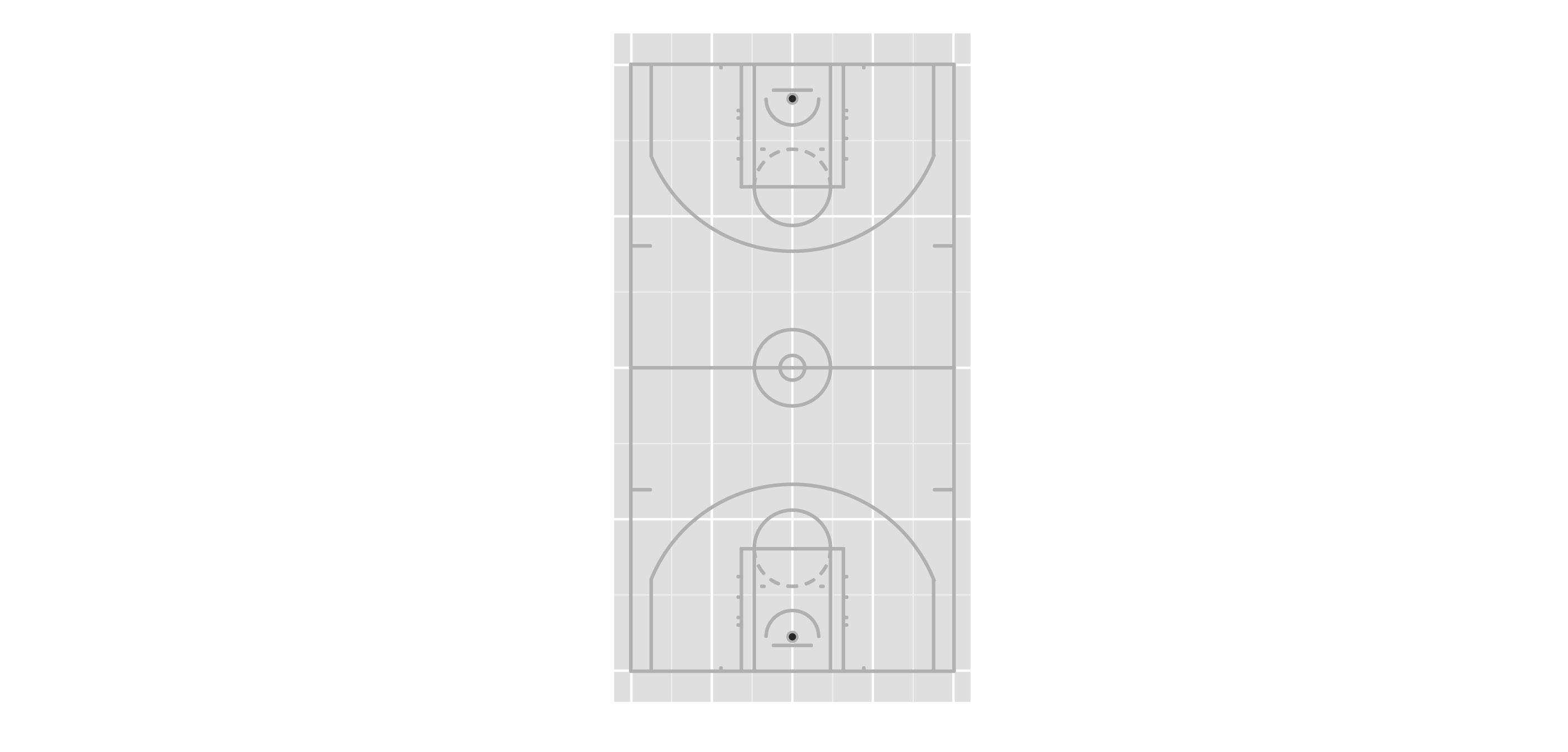Basketball court with ggplot2 (vertical). If we want to rotate the court, this function can be helpful: # Given the angle theta and the court data frame, # rotates the coordinates of the court by an angle theta rotate_court <- function(court, theta=pi/2){ court_r <- court court_r$x <- court_r$x / 180 * pi court_r$y <- court_r$y / 180 * pi matrice_r <- matrix(c(cos(theta), sin(theta), -sin(theta), cos(theta)), ncol = 2) coords_r <- apply(court_r[,c("x","y")], 1, function(x) x %*% matrice_r) court_r$x <- coords_r[1,] ; court_r$y <- coords_r[2,] court_r$x <- court_r$x * 180 / pi court_r$y <- court_r$y * 180 / pi return(court_r) }  # Whole court with rotation P_180 <- ggplot() + geom_polygon(data = rotate_court(court, theta = pi/2), aes(x = x, y = y, group = group), col = "gray") + coord_equal() + xlim(-2,96) + ylim(-55,2) + scale_x_continuous(breaks = c(0, 23.5, 47, 70.5, 94)) + scale_y_continuous(breaks = c(0, -12.5, -25, -37.5, -50)) + xlab("") + ylab("") + theme(axis.text.x = element_blank(), axis.text.y = element_blank(), axis.ticks.x = element_blank(), axis.ticks.y = element_blank(), axis.title = element_blank() ) P_180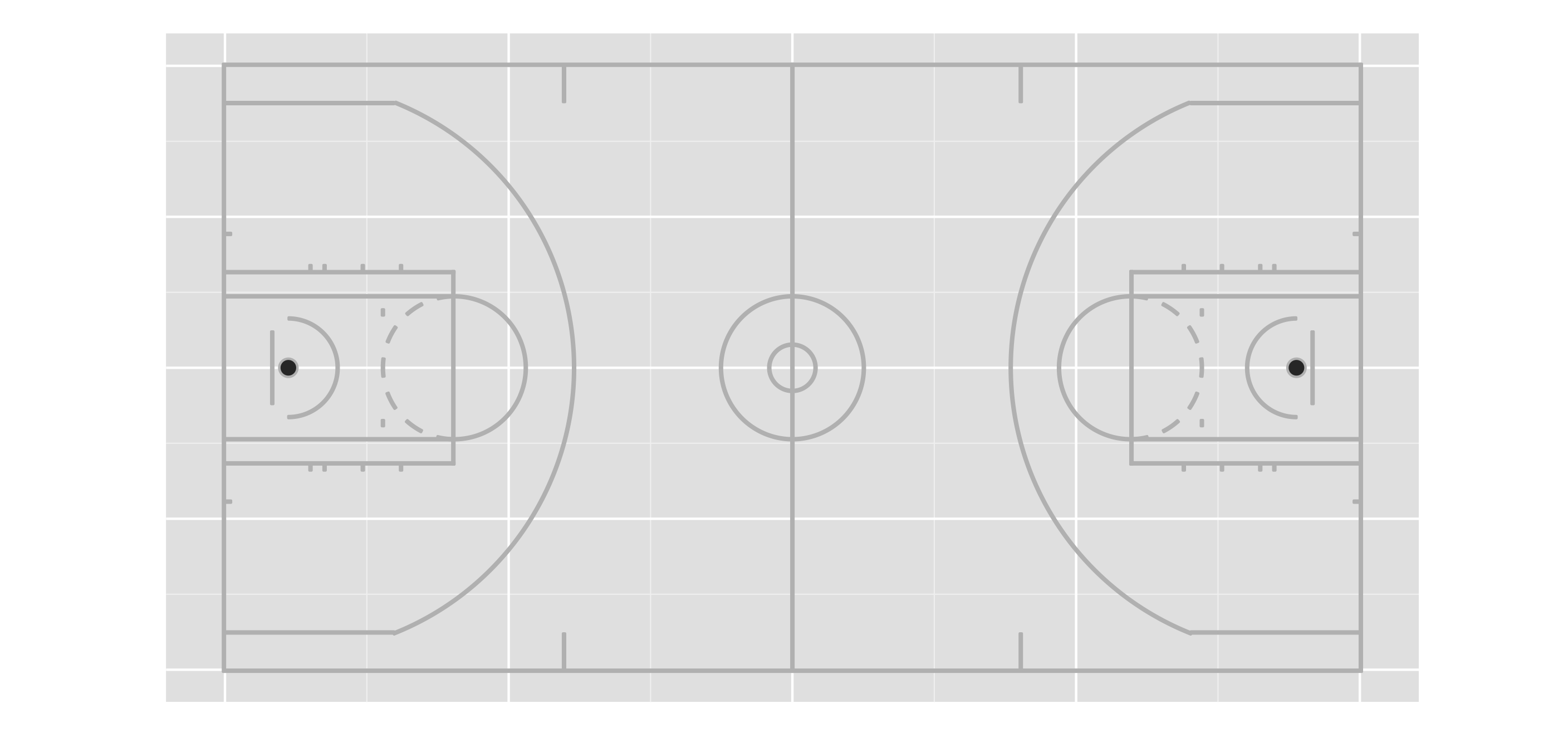Basketball court with ggplot2 (horizontal). It is also easy to get a half-court: P_half_180 <- ggplot() + geom_polygon(data = rotate_court(court[court$side==1,], theta = pi/2), aes(x = x, y = y, group = group), col = "gray") +
coord_equal() +
xlim(-2,50) +
ylim(-55,2) +
scale_x_continuous(breaks = c(0, 23.5, 47)) +
scale_y_continuous(breaks = c(0, -12.5, -25, -37.5, -50)) +
xlab("") + ylab("") +
theme(axis.text.x = element_blank(),
axis.text.y = element_blank(), axis.ticks.x = element_blank(),
axis.ticks.y = element_blank(), axis.title = element_blank()
)
P_half_180


To add points, we can create another data.frame with coordinates of the position of the player, and a dummy variable indicating wether the player scored or not.

shoots <- data.frame(x = c(2,4), y = c(2,10), score = c(TRUE,FALSE))
# If you use a rotate function, don't forget to apply the same rotation
# to your shoots coordinates !
shoots <- rotate_court(shoots, theta = pi/2)

P_half_180 + geom_point(data = shoots, aes(x = x, y = y, col = score), alpha = .8) +
scale_color_manual(values = c("TRUE" = "#00FF00", "FALSE" = "#FF0000"))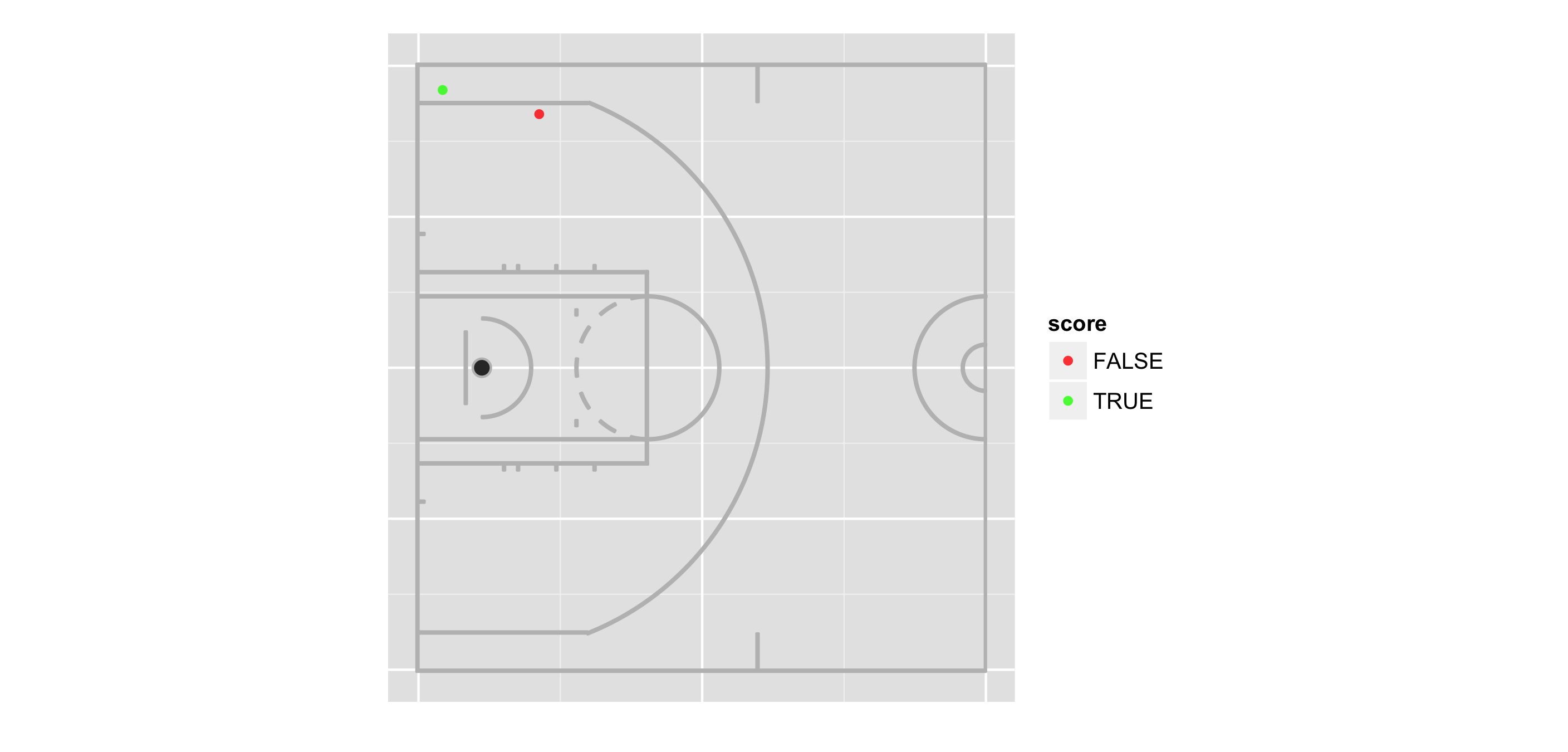Half-court with points.

Here is my attempt to recreate Andy Woodruff and Kirk Goldsberry’s animation, but this time, using R only.
Please note that it is easy to get a fixed court, after appending the following two lines to the plot:

xlim(-5,55) +
ylim(-5,55)


Also note that it is not that hard to plot the expected possession value on game clock, using “gtable” package.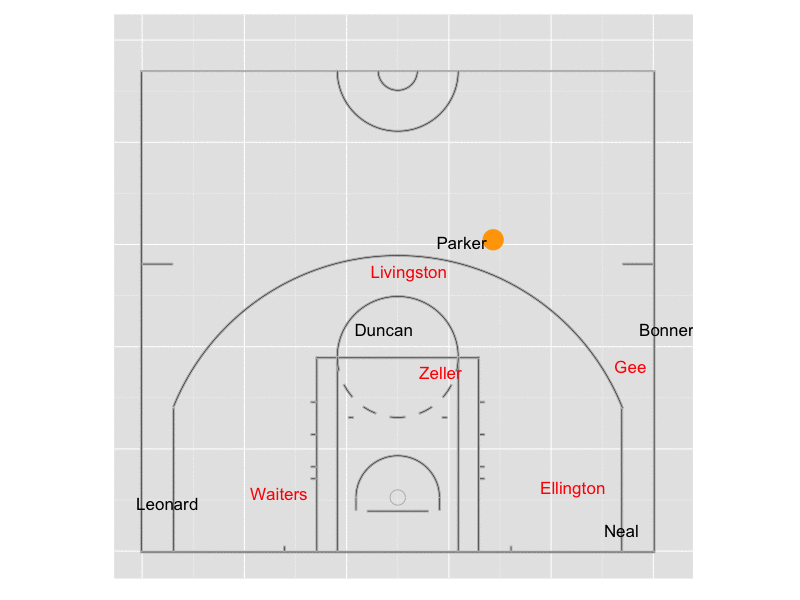Kawhi Leonard’s winning shot.

And to finish, let’s watch this play on YouTube:

[Update – March 2014]
Geotribu wrote a bit more on this topic on their weekly press (in French).

[Update – May 2014]
You can download the R file to draw either half or the whole court.

[Update – August 2014]
As requested in the comment section below (thank you AT), you can also download the R file to draw the college court.Basketball whole court with ggplot2.

## 6 thoughts on “Drawing a basketball court with R”

1.Mike says:

Hey there,

I just had a question that maybe you can help me with. I saw your post on recreating the Goldsberry NBA visuals by creating the court.

I’m just wondering if you could provide some help on overlaying a heatmap on top of this image that you create. I have data with x.coord, y.coord, pts (0, 2, or 3). I’m essentially trying to create a « heatmap » of points per attempt but overlayed on top of the court image you have provided the code for. In my ideal world, I’d love to place different sized circles/hexagons to represent frequency, and the shades to represent efficiency, but it seems like this is something hard to do in ggplot from what I’m reading.

Any help or tips on what ggplot functions you would use would be much appreciated!

-Mike

1.Ewen says:

Hi,

If you create a grid based on the court, you can easily estimate densities. You will get a three dimensional table (x,y,z). Then it is not really hard to do what you want, using geom_point function, where you can specify colors according to z, and shape according to the frequency for the (x,y) cell.
You might find this post useful to do so: Visualizing densities of spatial processes.

1.AT says:

Trying to copy your code into R to see if I can recreate this. I get this error that says it cannot find the function “qplot”. Anything you can think of for how I can get your code to work on R?

Thanks.

1.Ewen says:

Hello,
Have you loaded the “ggplot2” library? [code] library("ggplot2")[/code]. Be sure that ggplot2 is installed. If this is not the case, install it running this code: [code] install.packages("ggplot2") [/code].

I have improved the code to draw the whole court, I will upload the R file in a few minutes.

2.AT says:

Got it to work. Was wondering if you’d be able to help me draw a college basketball court representation. Please email me if possible.

1.Ewen says: